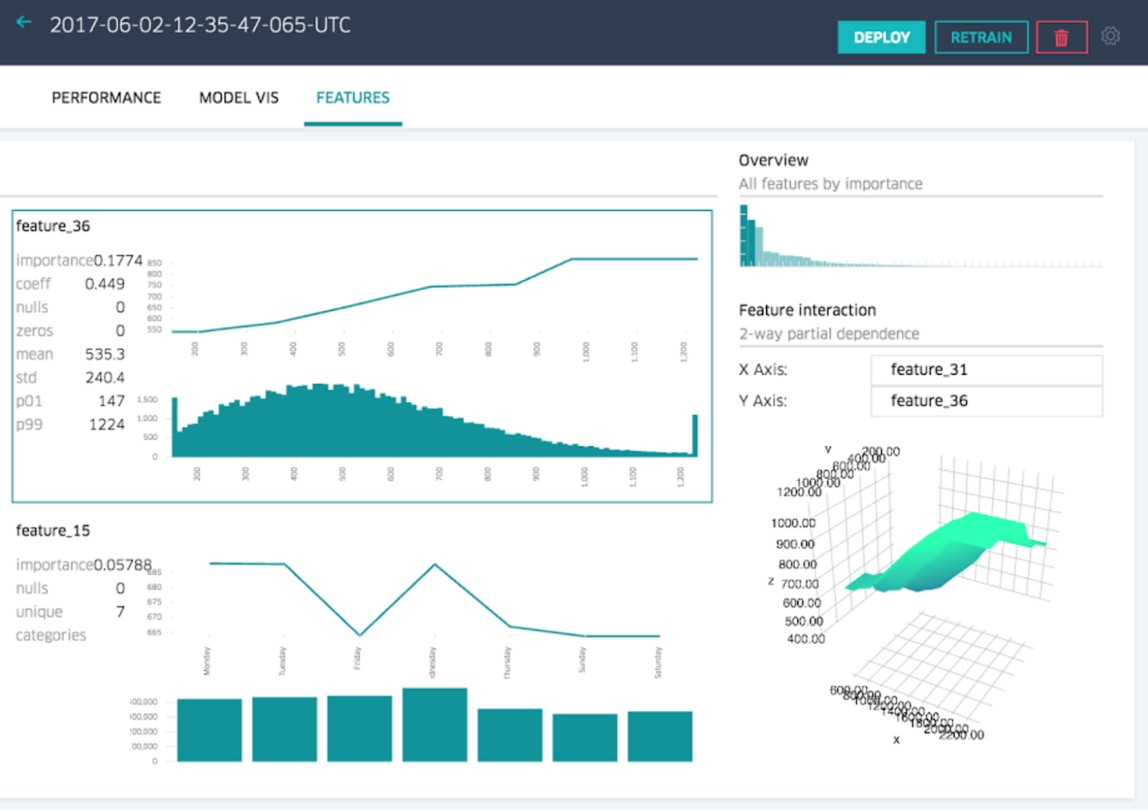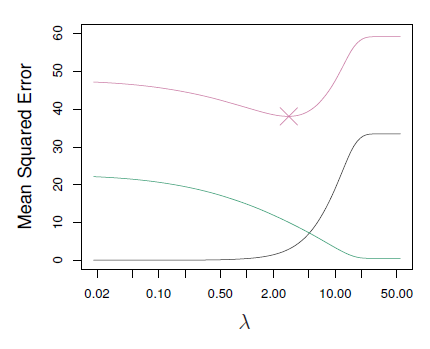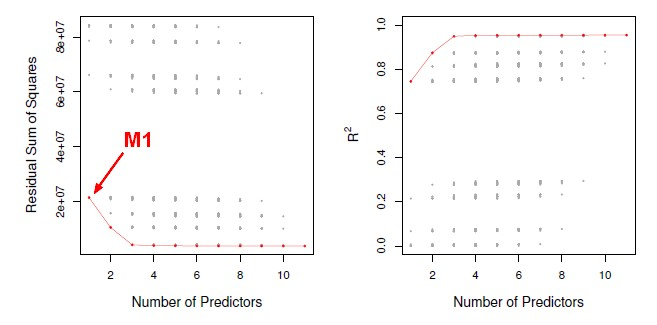# Data Mining - Model Size (d)

The model size (d) is the number of parameters.

In a regression model, the number of regression coefficient that are related to the number of feature.

Discover MoreData Mining - (Attribute|Feature) (Selection|Importance)

Feature selection is the second class of dimension reduction methods. They are used to reduce the number of predictors used by a model by selecting the best d predictors among the original p predictors....Data Mining - (Function|Model)

The model is the function, equation, algorithm that predicts an outcome value from one of several predictors. During the training process, the models are build. A model uses a logic and one of several...Data Mining - Dimensionality (number of variable, parameter) (P)

Not to confound with d: the model size. You may have 1000 attributes (p=1000) in your sample but after feature selection for instance, you model may use only a handful (d=5) In physics and mathematics,...Statistics - (Shrinkage|Regularization) of Regression Coefficients

Shrinkage methods are more modern techniques in which we don't actually select variables explicitly but rather we fit a model containingall p predictors using a technique that constrains or regularizes...Statistics - Model Selection

Model selection is the task of selecting a statistical model from a set of candidate models through the use of criteria's Dimension reduction procedures generates and returns a sequence of possible...